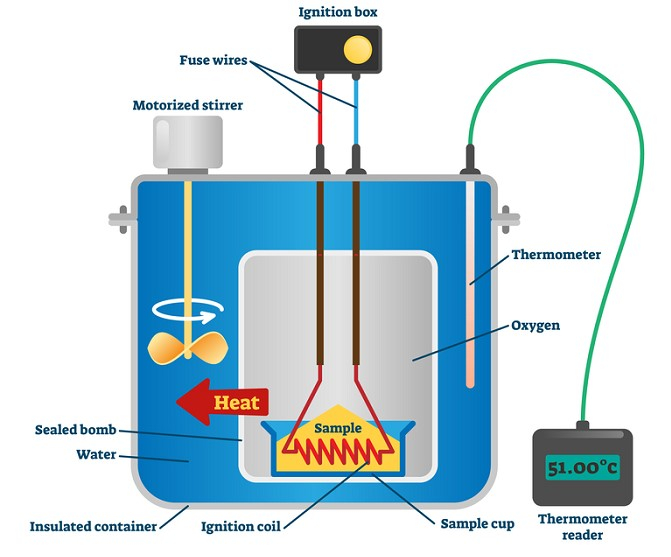# Measurement of Enthalpy and Internal Energy Change

## Introduction

Normally, the measurement of enthalpy and internal energy change is carried out by an experimental approach. This approach is known as calorimetry which is an important part of chemistry. It is mainly a technique which is developed on thermometric procedures. Here, a calorimeter is the vessel in which the procedure happens. In this part, the vessel is submerged in a known liquid volume.

## What is Enthalpy?

Enthalpy is the heat energy and it is absorbed during a chemical reaction progression. In this part, enthalpy is represented by H and it indicates the energy amount. The change of enthalpy is described through ΔH where delta refers to the change. It consists of a unit which is joules or kilojoules.

It can simply be described that the sum of internal energies of the system is enthalpy. The cause of this factor is that a change in internal energy takes place at the time of chemical reaction.

Here, the formula is

$\mathrm{H = U + PV}$

In this part, H is enthalpy, U is the sum of internal energy, P is the pressure of the system, and V is the volume of the system. Therefore, it is said that enthalpy is the addition of internal energy.

## What Is Internal Energy?

Internal energy is considered the addition of potential and kinetic energy of that particular system. In this part, the stored energy is potential energy which is released due to movement of molecules. It is the kinetic energy of the particle and internal energy is represented by U. The change in internal energy is described through the symbol ΔU.

The factors regarding this matter say that at a constant pressure internal energy and enthalpy are the same for a particular system. The change in this part can happen in two ways (Zhang, Shu & He, 2019). One happens because of the transfer of heat and the second happens because of doing work. The system can absorb or release heat and the change happened.

It is presented through the following equation:

$\mathrm{\Delta U=q +w}$

Here, ΔU is the internal energy change, q is the transfer of heat, and w is work done by or on a system.

## Enthalpy Change Measurement

The measurement of Enthalpy Change is described by expressing ΔH and it is the flow of heat at constant pressure. It is calculated by the use of a calorimeter of constant pressure. The factor shows the direct value of delta h enthalpy. The commercial calorimeters here also functioned on the similar principle.

They use the factor in a different way for describing the measurement of enthalpy change. The matter describes that a certain amount of energy is required for breaking the bond between one-mole molecules. The atomization enthalpy is needed for the conversion of any material intro gaseous atoms. Differently, it is said that sublimation enthalpy is the process where heat is essential for converting a mole of a substance from a solid to a gaseous state.

## Measurement of Change in Internal EnergyImage: Bomb calorimeter vector illustration - Constant volume type to measure heat internal energy

Internal energy change is referred to the change of energy at constant volume. For example, in this part, a bomb calorimeter is applied to measure internal energy change. This process used a steel vessel and it was seen as emerging in a water bath. It is happened considering the fact that no heat is lost to the surrounding.

It is observed that a combustible substance is mainly burnt in oxygen gas. The oxygen gas is supplied in the bomb here. It is seen that energy changes associated with the reaction. It can be measured in a constant volume because it does not change completely. The volume here remains constant and that is the reason the work is done at a zero system.

## Strategies

The strategies show different factors like

• It describes the calculation of a solution’s mass and its volume and density.

• The matter of evaluation of change in temperature of the solution is also described here.

• It determines heat’s flow.

• It uses KOH’s molar mass.

## Uses of Bomb Calorimeter

The Bomb Calorimeter is an important part of thermodynamics. It is seen that this type of calorimeter is utilised to decide heat release amount in burning reaction. It is also important for finding the calorific food value. Bomb calorimeters is most important for the industries and it is seen that it is mostly used in food processing, metabolic study, and testing of explosives.

It is very important for determining the heat which is emitted from a given quantity of biomass sample combustion. It is also used for calculating the HHV of that biomass fuel. It is considered to be an instrument for measuring heat. The instrument is very useful in calculating the calorific value of solids.

## Conclusion

The research shows that change in internal energy is mainly the sum of heat transmission and work done. The most important factor here is that internal energy and heat flow is related to the system’s enthalpy at constant pressure. Here, the heat is evaluated by using known heat capacities of the calorimeter and liquid. This mainly talks about experimental technique which is known as calorimetry.

## (FAQs)

Q1. What does enthalpy refer to?

Ans. Enthalpy is the released heat and it is absorbed during chemical reactions. In this part, H is the required energy and it presents the change in enthalpy. Here, delta represents the change and joules or kilojoules are used as units.

Q2. How does the measurement in the calorimetry process done?

Ans. Here, measurement is done with the use of two conditions and it is essential for this process. The conditions are at constant volume and at constant pressure. These are the most important part of the calorimetry process.